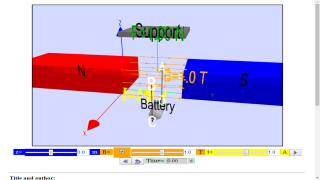Lorentz forces

## Lorentz force on a wire

This program simulates the force exerted by a magnetic field between two magnets on an electrical current trough a wire.

The wire is suspended on a spring and will oscillate when the battery (which is connected to the ends of the wire) is turned on and off, the angle of the wire with respect to the magnetic field is changed, or the poles of the magnets are switched.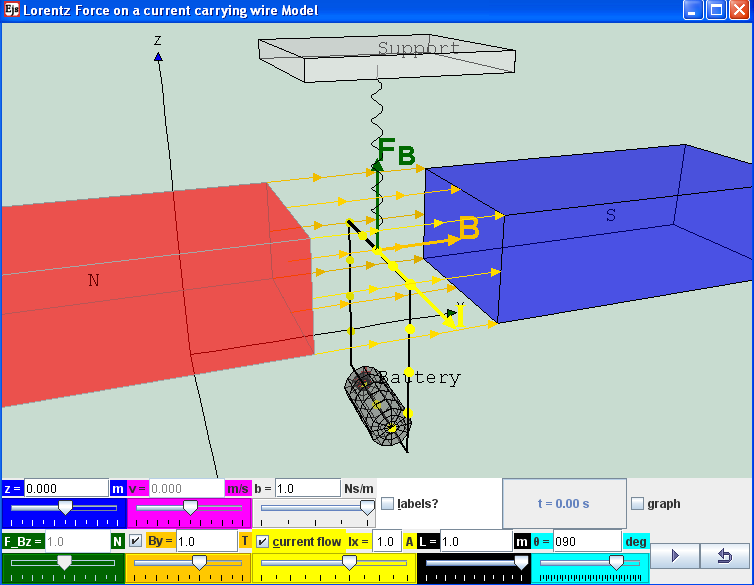new remixed version by lookang 20 Dec 2010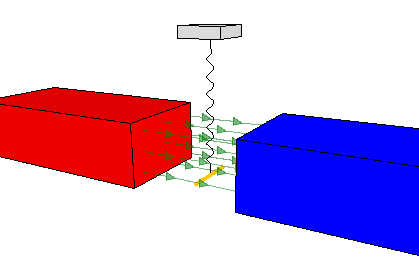original version by Professor Francisco (Paco) Esquembre

### Credits:

The Lorentz force on a wire model was created by Francisco (Paco) Esquembre, customized by Loo Kang Wee using the Easy Java Simulations (EJS) version 4.2 authoring and modeling tool.  An applet version of this model is available on the NTNU website < http://www.phy.ntnu.edu.tw/ntnujava/ >.

You can examine and modify this compiled EJS model if you run the model (double click on the model's jar file), right-click within a plot, and select "Open EJS Model" from the pop-up menu.  You must, of course, have EJS installed on your computer.  Information about EJS is available at: <http://www.um.es/fem/Ejs/> and in the OSP comPADRE collection <http://www.compadre.org/OSP/>.

Exercise Exercise by lookang

Introduction

www.bk.psu.edu/faculty/gamberg/mag_lab.doc
A current-carrying wire in a magnetic field experiences a force. The magnitude and direction of this force F, depend on four variables:

the magnitude and direction of the current (I),

the strength and direction of the magnetic field (B)
the length of the wire expose to magnetic field is (L)
the angle between the current I and field B is (ϑ)

Advanced: The force can be described mathematically by the vector cross-product:
O level: Fleming’s Left Hand Rule predicts the using the left hand, F (thumb) B (index finger) I (middle finger)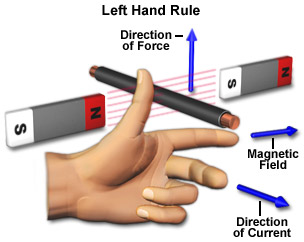image from National High Magnetic Field Laboratoryhttp://www.magnet.fsu.edu/education/tutorials/java/handrules/index.html

Advanced: F = I ^ B. L where ^ is the cross product

O level and A level: F = I . B. L.sin ϑ where ϑ is the angle between I and B

where
Force F is in newtons N
current I is in amperes A
length L in meters m
magnetic field B in teslas T

The direction of the force F is perpendicular to both the current I and the magnetic field B, and is predicted by the Advanced: right-hand cross-product rule.

O level and A level: Fleming’s Left Hand Rule

Engage:
a real live demo is the best.!!
Engage 1: Would you believe that a wire can jump up even though it is not alive?
Engage 2: have you thought about how a direct current can cause a rotating motion which can be used to drive some simple toys (e.g Tamiya cars) ?

http://www.tamiya.com/english/products/42183trf502x/top.jpg

Explore
1. Explore the simulation, this simulation is designed with a wire supported by a spring in a system of magnetic fields in y direction.
2 The play button runs the simulation, click it again to pause and the reset button brings the simulation back to its original state.
3 by default values B, I, L, play the simulation. Notice that the wire is in its motionless in its previous state of motion. What is the physics principle simulatted here.
hint: newton's 1st law
4 reset the simulation.
5 using the default values(L = 1 m, ϑ = 90 deg), adjust the value of By =1 and Ix =1 play the simulation. what did you observe? explain the motion in terms of the influences of magnetic field (assume gravitational effect can be neglected, in this computer model gravity is not model)
6 explore the slider z. what do this slider control?
7 explore the slider vz. what does this slider control?
8 by leaving the cursor on the slider, tips will appear to give a description of the slider. you can try it the following sliders such as the drag coefficient b.
9 there are some value of time of simulation t and the checkbox graph for height vs time.

10 vary the simulation and get a sense of what it does.

11 reset the simulation
Mechanics
12 using the default values (By =0, Ix=0) set z = -0.6, vz=0, b=0). Observe the motion of the wire in the absence of magnetic field. Predict what you will see. Describe the motion of the wire. Explain why this it is so?
hint: select the checkbox to view the scientific graph of height vs t.
13 using the default values (By =0, Ix=0) set z = -0.6, vz=0, b=1). Observe the motion of the wire in the absence of magnetic field. Predict what you will see. Describe the motion of the wire. Explain why this it is so?
hint: select the checkbox to view the scientific graph of height vs t.
14 using the default values (By =0, Ix=0) set z = -0.6, vz=1, b=0). Observe the motion of the wire in the absence of magnetic field. Predict what you will see. Describe the motion of the wire. Explain why this it is so?
hint: select the checkbox to view the scientific graph of height vs t.
15 using the default values (By =0, Ix=0) set z = -0.6, vz=1, b=1). Observe the motion of the wire in the absence of magnetic field. Predict what you will see. Describe the motion of the wire. Explain why this it is so?
hint: select the checkbox to view the scientific graph of height vs t.
16 conduct more scientific inquiry into the simulation if need before the next part of the question.
Elaborate

17 explain the effects of b, the model used is drag force = b.v.

18 reset the simulation
Magnetic Force
Evaluate:
19 A scientist hypothesis "O level and A level: F = I . B. L. where ϑ =90 deg" play the simulation for different initial condition and design an experiment with tables of values to record systematically, determine whether the hypothesis is accurate.

20 what is the impact of the ϑ != 90 deg ?
21 Suggest a better hypothesis
22 This computer model does not build in gravity, suggest with reason(s) why you agree or disagree with this statement. You can examine and modify this compiled EJS model if you run the model (double click on the model's jar file), right-click within a plot, and select "Open EJS Model" from the pop-up menu.  You must, of course, have EJS installed on your computer.  Information about EJS is available at:
and in the OSP comPADRE collection

Have Fun!
Lorentz force on a wire

### Translations

Code Language Translator Run### Software Requirements

SoftwareRequirements

 Android iOS Windows MacOS with best with Chrome Chrome Chrome Chrome support full-screen? Yes. Chrome/Opera No. Firefox/ Samsung Internet Not yet Yes Yes cannot work on some mobile browser that don't understand JavaScript such as..... cannot work on Internet Explorer 9 and below

### CreditsFrancisco (Paco) Esquembre; lookang (This email address is being protected from spambots. You need JavaScript enabled to view it.)

### end faq

http://iwant2study.org/lookangejss/05electricitynmagnetism_21electromagnetism/ejss_model_LorentzForcewee01/LorentzForcewee01_Simulation.xhtml

### Sample Learning Goals

O level: Fleming’s Left Hand Rule predicts the using the left hand, F (thumb) B (index finger) I (middle finger)

A level

A current-carrying wire in a magnetic field experiences a force. The magnitude and direction of this force F, depend on four variables:
the magnitude and direction of the current (I),
the strength and direction of the magnetic field (B)
the length of the wire expose to magnetic field is (L)
the angle between the current I and field B is (ϑ)
Advanced: The force can be described mathematically by the vector cross-product:

### For Teachers

This program simulates the force exerted by a magnetic field between two magnets on an electrical current trough a wire.
The wire is kept in equilibrium in the absence of gravity, suspended on a spring and will oscillate when the battery (which is connected to the ends of the wire) is turned on and off, the angle of the wire with respect to the magnetic field is changed, or the poles of the magnets are switched.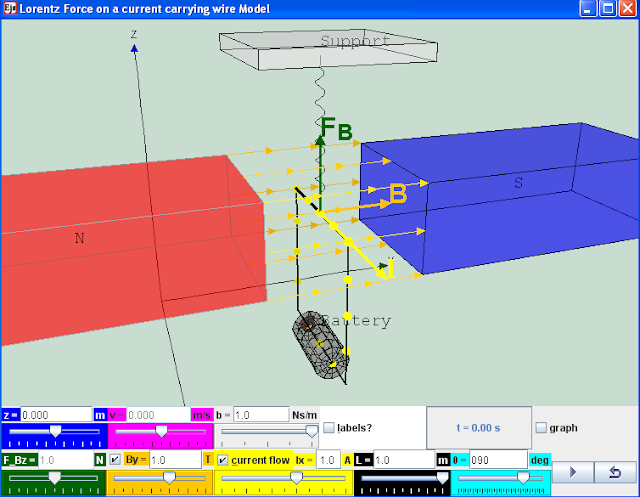reference:http://www.walter-fendt.de/ph14e/lorentzforce.htm

### Introduction

A current-carrying wire in a magnetic field experiences a force. The magnitude and direction of this force F, depend on four variables:
the magnitude and direction of the current (I),
the strength and direction of the magnetic field (B)
the length of the wire expose to magnetic field is (L)
the angle between the current I and field B is (ϑ)
Advanced: The force can be described mathematically by the vector cross-product:
O level: Fleming’s Left Hand Rule predicts the using the left hand, F (thumb) B (index finger) I (middle finger)
image from National High Magnetic Field Laboratory http://www.magnet.fsu.edu/education/tutorials/java/handrules/index.html

Advanced: F = I ^ B. L where ^ is the cross product
O level and A level: F = I . B. L.sin ϑ where ϑ is the angle between I and B

where
Force F is in newtons N
current I is in amperes A
length L in meters m
magnetic field B in teslas T

The direction of the force F is perpendicular to both the current I and the magnetic field B, and is predicted by the Advanced: right-hand cross-product rule.
O level and A level: Fleming’s Left Hand Rule

### Engage

Engage:
a real live demo is the best.!!

Engage 1: Would you believe that a wire can jump up even though it is not alive?
Engage 2: have you thought about how a direct current can cause a rotating motion which can be used to drive some simple toys (e.g Tamiya cars) ?
http://www.tamiya.com/english/products/42183trf502x/top.jpg

### Explore

1. Explore the simulation, this simulation is designed with a wire supported by a spring in a system of magnetic fields in y direction.
2 The play button runs the simulation, click it again to pause and the reset button brings the simulation back to its original state.
3 by default values B, I, L, play the simulation. Notice that the wire is in its motionless in its previous state of motion. What is the physics principle simulatted here.
hint: newton's 1st law
4 reset the simulation.
5 using the default values(L = 1 m, ϑ = 90 deg), adjust the value of By =1 and Ix =1 play the simulation. what did you observe? explain the motion in terms of the influences of magnetic field (assume gravitational effect can be neglected, in this computer model gravity is not model)
6 explore the slider z. what do this slider control?
7 explore the slider vz. what does this slider control?
8 by leaving the cursor on the slider, tips will appear to give a description of the slider. you can try it the following sliders such as the drag coefficient b.
9 there are some value of time of simulation t and the checkbox graph for height vs time.
10 vary the simulation and get a sense of what it does.

11 reset the simulation
Mechanics
12 using the default values (By =0, Ix=0) set z = -0.6, vz=0, b=0). Observe the motion of the wire in the absence of magnetic field. Predict what you will see. Describe the motion of the wire. Explain why this it is so?
hint: select the checkbox to view the scientific graph of height vs t.
13 using the default values (By =0, Ix=0) set z = -0.6, vz=0, b=1). Observe the motion of the wire in the absence of magnetic field. Predict what you will see. Describe the motion of the wire. Explain why this it is so?
hint: select the checkbox to view the scientific graph of height vs t.
14 using the default values (By =0, Ix=0) set z = -0.6, vz=1, b=0). Observe the motion of the wire in the absence of magnetic field. Predict what you will see. Describe the motion of the wire. Explain why this it is so?
hint: select the checkbox to view the scientific graph of height vs t.
15 using the default values (By =0, Ix=0) set z = -0.6, vz=1, b=1). Observe the motion of the wire in the absence of magnetic field. Predict what you will see. Describe the motion of the wire. Explain why this it is so?
hint: select the checkbox to view the scientific graph of height vs t.
16 conduct more scientific inquiry into the simulation if need before the next part of the question.
Elaborate
17 explain the effects of b, the model used is drag force = b.v.

18 reset the simulation
Magnetic Force
Evaluate:
19 A scientist hypothesis "O level and A level: F = I . B. L. where ϑ =90 deg" play the simulation for different initial condition and design an experiment with tables of values to record systematically, determine whether the hypothesis is accurate.

20 what is the impact of the ϑ != 90 deg ?
21 Suggest a better hypothesis
22 This computer model does not build in gravity, suggest with reason(s) why you agree or disagree with this statement. You can examine and modify this compiled EJS model if you run the model (double click on the model's jar file), right-click within a plot, and select "Open EJS Model" from the pop-up menu.  You must, of course, have EJS installed on your computer.  Information about EJS is available at: and in the OSP comPADRE collection

Have Fun!

Research

[text]

### Video

Electromagnetism (part 1): Force acting on a current-carrying conductor in a magnetic field by ETDtogo https://www.youtube.com/watch?v=URgZQ76tV1Y